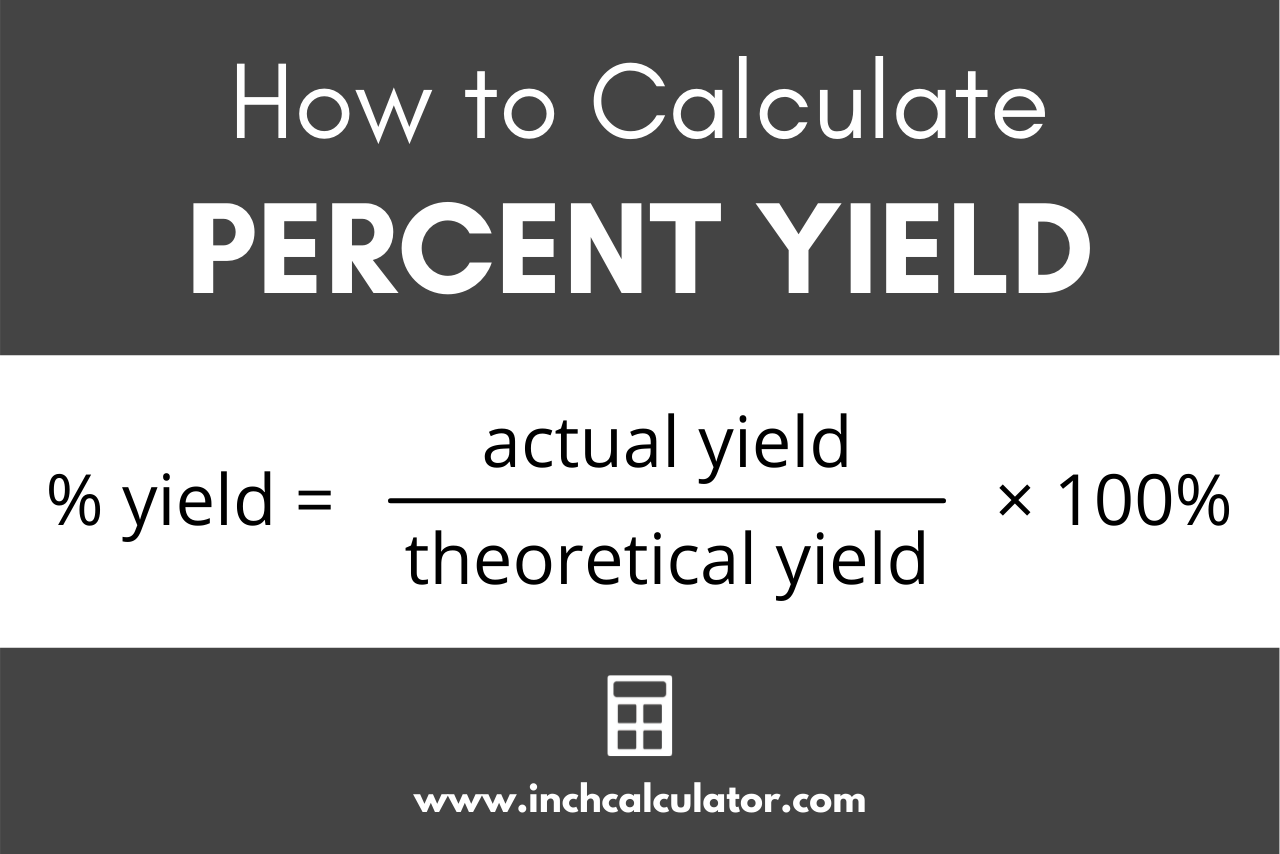# Percent Yield Calculator

Find percent yield, actual yield, and theoretical yield of a reaction using the yield calculator below. Simply select which value you want to find.

%
%

%

## How to Calculate Percent Yield

Percent yield is the ratio of the actual yield of a chemical reaction to the theoretical yield, expressed as a percentage.

Because a reaction is rarely 100% efficient, the resulting mass of the product after a reaction is rarely equal to the calculated yield. This occurs when the limiting reagent is not reacted completely or when other reactions occur in the experiment.

Percent yield is the comparison of the actual yield to the predicted theoretical yield. We can calculate percent yield using a standard formula.### Percent Yield Formula

Recalling that the percentage yield is a ratio of the actual yield to the theoretical yield, the percent yield formula can be stated as:

percent yield = actual yieldtheoretical yield × 100%

Percent yield is equal to the actual mass divided by the theoretical, or calculated mass, multiplied by 100%. Ensure that the inputs in the formula are all in the same unit of mass and convert them if necessary.

If you’re measurements for yields are in moles, you can also use a grams to moles calculator to convert to mass.

For example, let’s calculate the percent yield of a reaction with a theoretical yield of 4.85 g of product, but an actual yield of 4.47 g.

Apply the formula:

% yield = 4.47 g4.85 g × 100%
% yield = .922 × 100%
% yield = 92.2%

So, in this example, 92.2% is the percent yield, which is considered excellent.

## How to Calculate Percent Error

The amount of difference between the observed, or actual yield and the calculated, or theoretical yield is the percent error. You can calculate the error percentage by using our percent error calculator or with the formula below.

### Percent Error Formula

The percent error formula states:

percent error = |theoretical yield – actual yield|theoretical yield × 100%

The percentage of error is equal to the absolute value of the theoretical yield minus the actual yield, divided by the theoretical yield.

For example, let’s calculate the percent error of the reaction above with a theoretical yield of 4.85 g and an actual yield of 4.47 g.

Apply the formula:

% error = |4.85 g – 4.47 g|4.85 g × 100%
% error = 0.38 g4.85 g × 100%
% error = 0.078 × 100%
% error = 7.8%

So, in the example above, the percent error is 7.8%.

A keen observer might have noticed that the percent yield of 92.2% added with the percent error of 7.8% equals exactly 100%.

So we might simplify the concept of percent error to be 100% minus the percent yield, or basically the remaining reactant that was not reacted in the experiment.

## Why is Percent Yield Important?

Percent yield is a very important consideration in commercial and industrial chemistry. Since it’s a direct measure of the efficiency of a reaction, it is an important indicator of the amount of reactant required to make an actual product.

The goal of a chemist working on improving a reaction will be to improve the yield.

You might be wondering what a good percent yield value might be. Over 90% yield is considered excellent, 80-90% is deemed very good, 70-80% is good, 50-70% is fair, and below 40% is poor.

## How to Find the Actual & Theoretical Yield

You can also calculate the actual and theoretical yield by modifying the percent yield formula above to derive the following formulas.

### Actual Yield Formula

actual yield = percent yield100% × theoretical yield

The calculator above uses this equation to solve the actual yield given these inputs.

### Theoretical Yield Formula

theoretical yield = actual yieldpercentage yield × 100%

Of course, you can also use our theoretical yield calculator to find the calculated yield of a reaction.

## References

1. Ramsden, E.N., Calculations for A-level Chemistry, Thornes, 1995, 38.
2. LibreTexts, Percent Error, 2021, https://chem.libretexts.org/@go/page/52697
3. Furniss, B. S., Hannaford, A. J., Smith, P. W. G., Tatchell, A. R., Vogel's Textbook of Practical Organic Chemistry - Fifth Edition, Longman Scientific & Technical, 1989, 33-34.
4. Wink, D.J., Fetzer-Gislason, S., McNicholas, S., The Practice of Chemistry, W.H. Freeman, 2003, 328.